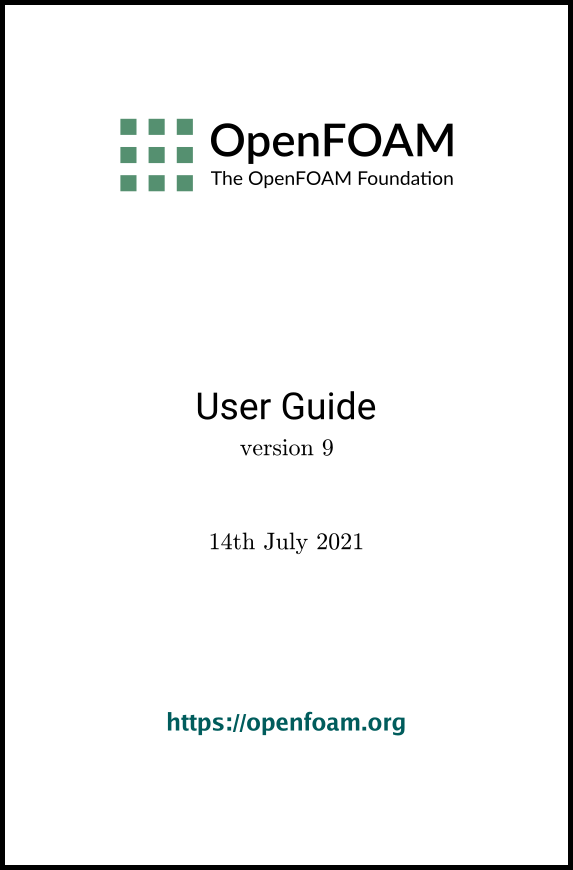## 7.2Turbulence models

The momentumTransport dictionary is read by any solver that includes turbulence modelling. Within that ﬁle is the simulationType keyword that controls the type of turbulence modelling to be used, either:

laminar
uses no turbulence models;
RAS
uses Reynolds-averaged simulation (RAS) modelling;
LES
uses large-eddy simulation (LES) modelling.

### 7.2.1Reynolds-averaged simulation (RAS) modelling

If RAS is selected, the choice of RAS modelling is speciﬁed in a RAS sub-dictionary which requires the following entries.

• model: name of RAS turbulence model.
• turbulence: switch to turn the solving of turbulence modelling on/oﬀ.
• printCoeffs: switch to print model coeﬀs to terminal at simulation start up.
• <model>Coeffs: dictionary of coeﬃcients for the respective model, to override the default coeﬃcients.

Turbulence models can be listed by running a solver with the -listMomentumTransportModels option, e.g.

simpleFoam -listMomentumTransportModels
With simpleFoam, the incompressible models are listed. The compressible models are listed for a compressible solver, e.g. rhoSimpleFoam.

The RAS models used in the tutorials can be listed using foamSearch with the following command. The lists of available models are given in the following sections.

foamSearch \$FOAM_TUTORIALS momentumTransport RAS/model
Users can locate tutorials using a particular model, e.g. buoyantKEpsilon, using foamInfo.

foamInfo buoyantKEpsilon

#### 7.2.1.1Incompressible RAS turbulence models

For incompressible ﬂows, the RAS model can be chosen from the list below.

LRR
Launder, Reece and Rodi Reynolds-stress turbulence model for incompressible ﬂows.
LamBremhorstKE
Lam and Bremhorst low-Reynolds number k-epsilon turbulence model for incompressible ﬂows.
LaunderSharmaKE
Launder and Sharma low-Reynolds k-epsilon turbulence model for incompressible ﬂows.
LienCubicKE
Lien cubic non-linear low-Reynolds k-epsilon turbulence models for incompressible ﬂows.
LienLeschziner
Lien and Leschziner low-Reynolds number k-epsilon turbulence model for incompressible ﬂows.
RNGkEpsilon
Renormalization group k-epsilon turbulence model for incompressible ﬂows.
SSG
Speziale, Sarkar and Gatski Reynolds-stress turbulence model for incompressible ﬂows.
Shih’s quadratic algebraic Reynolds stress k-epsilon turbulence model for incompressible ﬂows
SpalartAllmaras
Spalart-Allmaras one-eqn mixing-length model for incompressible external ﬂows.
kEpsilon
Standard k-epsilon turbulence model for incompressible ﬂows.
kOmega
Standard high Reynolds-number k-omega turbulence model for incompressible ﬂows.
kOmega2006
Standard (2006) high Reynolds-number k-omega turbulence model for incompressible ﬂows.
kOmegaSST
Implementation of the k-omega-SST turbulence model for incompressible ﬂows.
kOmegaSSTLM
Langtry-Menter 4-equation transitional SST model based on the k-omega-SST RAS model.
kOmegaSSTSAS
Scale-adaptive URAS model based on the k-omega-SST RAS model.
kkLOmega
Low Reynolds-number k-kl-omega turbulence model for incompressible ﬂows.
qZeta
Gibson and Dafa’Alla’s q-zeta two-equation low-Re turbulence model for incompressible ﬂows
realizableKE
Realizable k-epsilon turbulence model for incompressible ﬂows.
v2f
Lien and Kalitzin’s v2-f turbulence model for incompressible ﬂows, with a limit imposed on the turbulent viscosity given by Davidson et al.

#### 7.2.1.2Compressible RAS turbulence models

For compressible ﬂows, the RAS model can be chosen from the list below.

LRR
Launder, Reece and Rodi Reynolds-stress turbulence model for compressible ﬂows.
LaunderSharmaKE
Launder and Sharma low-Reynolds k-epsilon turbulence model for compressible and combusting ﬂows including rapid distortion theory (RDT) based compression term.
RNGkEpsilon
Renormalization group k-epsilon turbulence model for compressible ﬂows.
SSG
Speziale, Sarkar and Gatski Reynolds-stress turbulence model for compressible ﬂows.
SpalartAllmaras
Spalart-Allmaras one-eqn mixing-length model for compressible external ﬂows.
buoyantKEpsilon
Additional buoyancy generation/dissipation term applied to the k and epsilon equations of the standard k-epsilon model.
kEpsilon
Standard k-epsilon turbulence model for compressible ﬂows including rapid distortion theory (RDT) based compression term.
kOmega
Standard high Reynolds-number k-omega turbulence model for compressible ﬂows.
kOmega2006
Standard (2006) high Reynolds-number k-omega turbulence model for compressible ﬂows.
kOmegaSST
Implementation of the k-omega-SST turbulence model for compressible ﬂows.
kOmegaSSTLM
Langtry-Menter 4-equation transitional SST model based on the k-omega-SST RAS model.
kOmegaSSTSAS
Scale-adaptive URAS model based on the k-omega-SST RAS model.
realizableKE
Realizable k-epsilon turbulence model for compressible ﬂows.
v2f
Lien and Kalitzin’s v2-f turbulence model for compressible ﬂows, with a limit imposed on the turbulent viscosity given by Davidson et al.

### 7.2.2Large eddy simulation (LES) modelling

If LES is selected, the choice of LES modelling is speciﬁed in a LES sub-dictionary which requires the following entries.

• model: name of LES turbulence model.
• delta: name of delta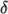model.
• <model>Coeffs: dictionary of coeﬃcients for the respective model, to override the default coeﬃcients.
• <delta>Coeffs: dictionary of coeﬃcients for the delta model.

The LES models used in the tutorials can be listed using foamSearch with the following command. The lists of available models are given in the following sections.

foamSearch \$FOAM_TUTORIALS momentumTransport LES/model

#### 7.2.2.1Incompressible LES turbulence models

For incompressible ﬂows, the LES model can be chosen from the list below.

DeardorﬀDiﬀStress
Diﬀerential SGS Stress Equation Model for incompressible ﬂows
Smagorinsky
The Smagorinsky SGS model.
SpalartAllmarasDDES
SpalartAllmaras DDES turbulence model for incompressible ﬂows
SpalartAllmarasDES
SpalartAllmarasDES DES turbulence model for incompressible ﬂows
SpalartAllmarasIDDES
SpalartAllmaras IDDES turbulence model for incompressible ﬂows
WALE
The Wall-adapting local eddy-viscosity (WALE) SGS model.
dynamicKEqn
Dynamic one equation eddy-viscosity model
dynamicLagrangian
Dynamic SGS model with Lagrangian averaging
kEqn
One equation eddy-viscosity model
kOmegaSSTDES
Implementation of the k-omega-SST-DES turbulence model for incompressible ﬂows.

#### 7.2.2.2Compressible LES turbulence models

For compressible ﬂows, the LES model can be chosen from the list below.

DeardorﬀDiﬀStress
Diﬀerential SGS Stress Equation Model for compressible ﬂows
Smagorinsky
The Smagorinsky SGS model.
SpalartAllmarasDDES
SpalartAllmaras DDES turbulence model for compressible ﬂows
SpalartAllmarasDES
SpalartAllmarasDES DES turbulence model for compressible ﬂows
SpalartAllmarasIDDES
SpalartAllmaras IDDES turbulence model for compressible ﬂows
WALE
The Wall-adapting local eddy-viscosity (WALE) SGS model.
dynamicKEqn
Dynamic one equation eddy-viscosity model
dynamicLagrangian
Dynamic SGS model with Lagrangian averaging
kEqn
One equation eddy-viscosity model
kOmegaSSTDES
Implementation of the k-omega-SST-DES turbulence model for compressible ﬂows.

### 7.2.3Model coeﬃcients

The coeﬃcients for the RAS turbulence models are given default values in their respective source code. If the user wishes to override these default values, then they can do so by adding a sub-dictionary entry to the RAS sub-dictionary ﬁle, whose keyword name is that of the model with Coeffs appended, e.g. kEpsilonCoeffs for the kEpsilon model. If the printCoeffs switch is on in the RAS sub-dictionary, an example of the relevant …Coeffs dictionary is printed to standard output when the model is created at the beginning of a run. The user can simply copy this into the RAS sub-dictionary ﬁle and edit the entries as required.

### 7.2.4Wall functions

A range of wall function models is available in OpenFOAM that are applied as boundary conditions on individual patches. This enables diﬀerent wall function models to be applied to diﬀerent wall regions. The choice of wall function model is speciﬁed through the turbulent viscosity ﬁeld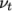in the 0/nut ﬁle. For example, a 0/nut ﬁle:

16
17dimensions      [0 2 -1 0 0 0 0];
18
19internalField   uniform 0;
20
21boundaryField
22{
23    movingWall
24    {
25        type            nutkWallFunction;
26        value           uniform 0;
27    }
28    fixedWalls
29    {
30        type            nutkWallFunction;
31        value           uniform 0;
32    }
33    frontAndBack
34    {
35        type            empty;
36    }
37}
38
39
40// ************************************************************************* //

There are a number of wall function models available in the release, e.g. nutWallFunction, nutRoughWallFunction, nutUSpaldingWallFunction, nutkWallFunction and nutkAtmWallFunction. The user can get the full list of wall function models using foamInfo:

foamInfo wallFunction
Within each wall function boundary condition the user can over-ride default settings for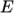,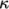and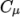through optional E, kappa and Cmu keyword entries.

Having selected the particular wall functions on various patches in the nut/mut ﬁle, the user should select epsilonWallFunction on corresponding patches in the epsilon ﬁeld and kqRwallFunction on corresponding patches in the turbulent ﬁelds k, q and R.

OpenFOAM v9 User Guide - 7.2 Turbulence models